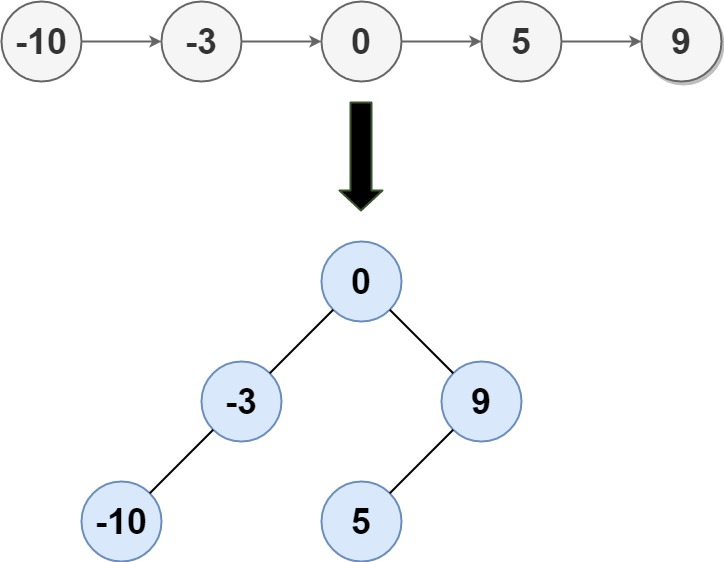109. Convert Sorted List to Binary Search Tree

Given the `head` of a singly linked list where elements are sorted in ascending order, convert it to a height balanced BST.

For this problem, a height-balanced binary tree is defined as a binary tree in which the depth of the two subtrees of every node never differ by more than 1.

Example 1:```Input: head = [-10,-3,0,5,9]
Output: [0,-3,9,-10,null,5]
Explanation: One possible answer is [0,-3,9,-10,null,5], which represents the shown height balanced BST.
```

Example 2:

```Input: head = []
Output: []
```

Example 3:

```Input: head = 
Output: 
```

Example 4:

```Input: head = [1,3]
Output: [3,1]
```

Constraints:

• The number of nodes in `head` is in the range `[0, 2 * 104]`.
• `-10^5 <= Node.val <= 10^5`

109. Convert Sorted List to Binary Search Tree
``````struct Solution;
use rustgym_util::*;

trait Convert {
fn count(&self) -> usize;
}

fn count(&self) -> usize {
let mut p = self;
let mut n = 0;
while let Some(node) = p {
p = &node.next;
n += 1;
}
n
}
if n == 0 {
return (None, self);
}
if n == 1 {
let mut node = self.unwrap();
return (tree!(node.val), node.next.take());
}
let (left, next) = self.convert(n / 2);
let (middle, next) = next.convert(1);
let (right, next) = next.convert(n - n / 2 - 1);
let node = middle.unwrap();
node.borrow_mut().left = left;
node.borrow_mut().right = right;
(Some(node), next)
}
}

impl Solution {